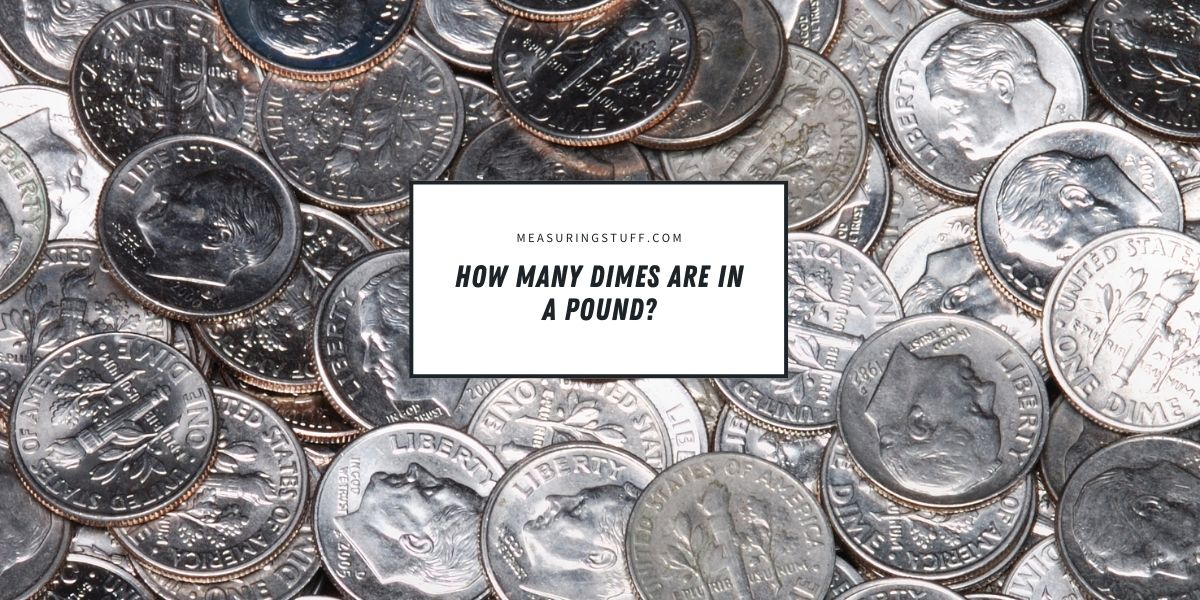# How Many Dimes Are In A Pound?

Dimes are such a common sight for people in the United States that we don’t often think too much about how they’re made or what they weigh. However, if you’re trying to figure out a math problem or you need to know how many dimes can fit in a certain container, finding out how many dimes there are in 1 pound can be both fascinating and helpful.

## How much does a dime weigh?

To figure out how many dimes are in 1 pound, we first need to know how much a single dime weighs. Although they are worth 10 cents each, which is worth more than both a single penny or a single nickel, dimes are the smallest United States coin.

Dimes are made with nickel and copper wrapped around a copper core. They are 17.91 millimeters or 0.705 inches in diameter and have a thickness of 1.35 millimeters or 0.05 inches. Their size and the metal they are made of give them a weight of 2.268 grams or about 0.08 ounces.

When they are minted, dimes weigh exactly 2.268 grams. However, this weight can change slightly over time, especially if the dime has been circulating for several years.

Some dimes see a good deal of wear, and any metal that has been smoothed away will slightly reduce the weight of the coin. Similarly, dimes that have a build-up of dirt can weigh slightly more.

These fluctuations in weight will be very minor, however, and they may sometimes even cancel each other out.

## How many dimes are in 1 pound?

There are a couple of ways to find out roughly how many dimes are in 1 pound. First, we can use the more exact weight of a single dime, which is 2.268 grams. There are 28.3495 grams in 1 ounce. If we divide 28.3495 by 2.268, we find that it would take about 12.5 dimes to make up 1 ounce.

There are 16 ounces in 1 pound. We can multiply 12.5 by 16 to find that there are about 200 dimes in 1 pound.

CHECK OUT  How Thick Is A Stack Of 100 Dollar Bills?

We can also use a dime’s weight in ounces to reach an answer. A dime weighs about 0.08 ounces. If we divide 1 by 0.08, we get 12.5. We then multiply by 16 to again get 200.

Using both weights helps us check our math and can show us if there are any discrepancies due to the rounding of weights when converting between grams and ounces.

## How much is 1 pound of dimes worth?

One dime is worth 10 cents, or \$0.10. We can find out how much 1 pound of dimes is worth by multiplying 200, which is the number of dimes in 1 pound, by 0.1. The answer is 20, which tells us that 1 pound of dimes is worth \$20.

## How many dimes are in a roll?

People often roll dimes or other coins. These rolls of coins can then be turned in at the bank for dollar bills, which are more portable, or deposited into a bank account. Banks require that coins are rolled in specific wrappers, and each wrapper holds a specific amount of coins so they can be counted quickly and accurately.

There are 50 dimes in one standard roll. We know that a dime is worth 10 cents, so we can multiply 0.1 by 50 to find that a roll of dimes is worth \$5.

You can also figure out how much a roll of dimes weighs by using the same numbers from above. A dime weighs 2.268 grams, which we can multiply by 50. The answer, which is 113.4, tells us that a roll of dimes weighs 113.4 grams, not including the wrapper. There are 28.3495 grams in an ounce. By dividing 28.3495 by 113.4, we can find that one roll of dimes is equal to almost exactly 4 ounces. This is about 1/4 of a pound.

## What is the history of the dime?

The dime was first introduced in the United States in 1796 after a coin representing 1/10 of a dollar was proposed by Alexander Hamilton. Until 1837, dimes were made mostly of silver, but this led to a high production cost. In 1965, silver dimes were officially put out of production, and today’s copper and nickel dimes were introduced.